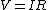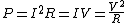# electrical resistance

(redirected from Resistance (electricity))
Also found in: Dictionary, Thesaurus.

## Electrical resistance

Opposition of a circuit to the flow of electric current. Ohm's law states that the current I flowing in a circuit is proportional to the applied potential difference V. The constant of proportionality is defined as the resistance R. Hence, Eq. (1)

(1)holds. If V and I are measured in volts and amperes, respectively, R is measured in ohms. Microscopically, resistance is associated with the impedance to flow of charge carriers offered by the material. For example, in a metallic conductor the charge carriers are electrons moving in a polycrystalline material in which their journey is impeded by collisions with imperfections in the local crystal lattice, such as impurity atoms, vacancies, and dislocations. In these collisions the carriers lose energy to the crystal lattice, and thus Joule heat is liberated in the conductor, which rises in temperature. The Joule heat P is given by
(2)Eq. (2). See Crystal defects, Electrical resistivity, Joule's law, Ohm's law

## electrical resistance

[i′lek·trə·kəl ri′zis·təns]
(acoustics)

## electrical resistance

The physical property of a device, conductor, element, branch, or system, by virtue of which power is lost as heat when current flows through it; the physical property which an electric conductor exhibits to the flow of current; measured in ohms.
Site: Follow: Share:
Open / Close